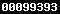### EKSPERIMENTASI MODEL PEMBELAJARAN KOOPERATIF TIPE TEAM ASSISTED INDIVIDUALIZATION GUIDE NOTE TAKING (TAI GNT) DITINJAU DARI KEMANDIRIAN BELAJAR SISWA

#### Sari

Abstract: The aim of the research was to determine the effect of learning models on mathematics learning achievement viewed from students independence learning. The learning models compared were cooperative learning model of TAI GNT, cooperative learning model of TAI, and conventional model. The type of the research was a quasi-experimental research. The population was the tenth grade students of senior high school at East Lombok in the first semester of the academic year 2012/2013. The size of the sample was 104 students is given the cooperative learning model of TAI GNT type, 106 students is given the cooperative learning model of TAI type, 105 students is given model of conventional type. The instruments used were documentation, questionnaire, and test methods. The data was analyzed using two way analysis of variance. The results of this research are as follows. (1) The TAI GNT model gives better mathematics learning achievement than TAI and conventional model, besides, TAI model gives better mathematics learning achievement than conventional model. (2) The students with high independence learning have better mathematic learning achievement than students with medium and low independence learning, and the students with medium independence learning have the same mathematics learning achievement as the students with low independence learning. (3) In each level of independence learning (high, medium, and low), TAI GNT model gives better mathematics learning achievement than TAI and conventional model, besides, TAI model gives better mathematic learning achievement than conventional model. (4) In each learning models (TAI GNT, TAI and conventional), the students with high independence learning have better mathematics learning achievement than the students with medium and low independence learning, and the students with medium independence learning have the same mathematics learning achievement as the students with low independence learning.

Keywords: cooperative learning, team assisted individualization, guide note taking, independence learning.

PDF

### Refbacks

• Saat ini tidak ada refbacks.View My Stats